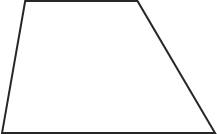# Trapezoid Area Calculator

If you are wondering how to find the area of trapezoids, you’re in the right place – this area of a trapezoid calculator is a tool for you. Whether you are looking for a calculation tool or want to know more about the formula behind the calculator, we’ve got you covered. Scroll down to find out more about the trapezoid area formula or give our calculator a try.

## What is a trapezoid?

A trapezoid is a 4-sided flat shape with straight sides that has a pair of opposite sides parallel.## Trapezoid area formula

Area of a trapezoid is found according to the following formula:

`area = (b1 + b2) * h / 2`

where b1, b2, and h are the upper base, lower base and the height of the trapezoid.

## How to find the area of a trapezoid?

You can use the trapezoid area calculator to find the area of a trapezoid:

1. Enter the length of the two bases (b1 and b2) of the trapezoid.
2. Enter the height.
3. Our trapezoid area calculator performs all the calculations for you and you can now get the result at the bottom of the calculator.

#### This calculator is created with

the best online spreadsheet editor with excellent formula and editing capability.

For more calculators for finance, mathematics health, unit converters and more check out our calculators collection.